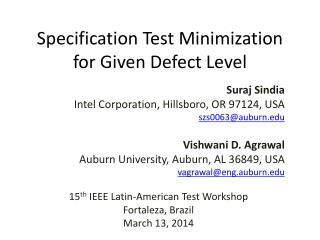DownloadDownload PresentationSpecification Test Minimization for Given Defect Level

# Specification Test Minimization for Given Defect Level

Download Presentation## Specification Test Minimization for Given Defect Level

- - - - - - - - - - - - - - - - - - - - - - - - - - - E N D - - - - - - - - - - - - - - - - - - - - - - - - - - -
##### Presentation Transcript

1. Specification Test Minimization for Given Defect Level Suraj Sindia Intel Corporation, Hillsboro, OR 97124, USA szs0063@auburn.edu Vishwani D. Agrawal Auburn University, Auburn, AL 36849, USA vagrawal@eng.auburn.edu 15th IEEE Latin-American Test Workshop Fortaleza, Brazil March 13, 2014

2. Problem Statement • Given a set of complete specification-based tests for an analog or RF circuit, and • An acceptable defect level (DL), • Find the smallest set of tests that should be used. LATW 2014: Spec. Test Minimization

3. Motivation International Technology Roadmap for Semiconductors (ITRS) 2009 http://www.itrs.net/Links/2009ITRS/Home2009.htm LATW 2014: Spec. Test Minimization

4. What is Defect Level? Good chip Bad chip Tested good Defect level: DL = 2/21 Yield loss: YL = 1/30 Tested bad True yield: Y = 20/30 All fabricated chips LATW 2014: Spec. Test Minimization

5. Definitions and Assumption • Specification Si is tested by test Ti. • Probability of testing Sjby Ti Is pij. • Assume that specification tests have zero defect level: • p11 = p22 = ● ● ●= 1.0 • This is perhaps the reason why the users and manufacturers of VLSI have more confidence in specification tests than in alternate tests. • This assumption can be relaxed in the future work. LATW 2014: Spec. Test Minimization

6. A Bipartite Graph Tests T1 T2 T3 T4 p12 p33 p13 p11 p44 p22 p42 p21 p34 S2 S1 S3 S4 Specifications LATW 2014: Spec. Test Minimization

7. An Integer Linear Program (ILP) • Consider k specifications and k tests. • Define k integer [0,1] variables {xi} for tests {Ti }: • Discard Tiif xi= 0, else retain Ti • Define objective function: k minimize ∑ xi i=1 • Next, need linear constraints to stay within given defect level. LATW 2014: Spec. Test Minimization

8. Defect Level: A Faulty Device Passes • Defect level is probability of a faulty device passing all tests, i.e., Prob{All tests pass | device is faulty} • For given defect level (dl), this conditional probability should not exceed dl, i.e., k 1 – ∏ P(Sj) ≤ dl j=1 • Where, P(Sj) = Probability of testing specification Sj k = 1 – ∏ (1 – pij)xi i=1 LATW 2014: Spec. Test Minimization

9. Giving Equal Weight per Specification • Assume that each specification weighs equally in determining defect level, P(S1) = P(S2) = ● ● ● =P(Sk) or 1 – [P(Sj)]k ≤ dl or (1 – dl)1/k ≤ P(Sj), j = 1, 2, ● ● ● , k k or (1 – dl)1/k ≤ P(Sj) = 1 – ∏ (1 – pij)xi i=1 j = 1, 2, ● ● ● , k LATW 2014: Spec. Test Minimization

10. Linear Constraints • We derive k linear constraint relations for variables xi and constant dl: k (1 – dl)1/k ≤ 1 – ∏ (1 – pij)xi,j = 1, 2, ● ● ● , k i=1 Therefore, k ∑ xiln(1 – pij) ≤ ln[1 – (1 – dl)1/k], i=1 j = 1, 2, ● ● ● , k LATW 2014: Spec. Test Minimization

11. Operational Amplifier: TI LM741 LATW 2014: Spec. Test Minimization

12. LM741 Specifications LATW 2014: Spec. Test Minimization

13. Monte Carlo Simulation • Simulate sample circuits for tests T1 through T7 using spice. • 5,000 circuit samples generated: • 5% random deviation around nominal value of each components (12 resistors and 1 capacitor) • 10% random deviation in DC gain of each BJT LATW 2014: Spec. Test Minimization

14. Compute probabilities pij • X = circuits failing Ti • Y = circuits failing Tj • Z = circuits failing both Tiand Tj • pij= Prob{Test Tj fails | spec Si is faulty} = Z/Y • Example: • 45 circuits had spec. S1 failure, detected by T1 • 81 circuits had spec. S2 failure, detected by T2 • 17 circuits had both failures • p12 = 17/81 = 0.21, p21 = 17/45 = 0.38, p11 = p22 = 1.0 LATW 2014: Spec. Test Minimization

15. Spice Simulation of 5,000 Samples Samples failing T1 p12 = 17/81 = 0.21 p21 = 17/45 = 0.38 LATW 2014: Spec. Test Minimization

16. Probabilities pij for LM741 LATW 2014: Spec. Test Minimization

17. ILP • Define xi [0,1], such that xi = 0  discard Ti. • Objective function: 7 minimize ∑ xi i=1 • Subject to: 7 ∑ xiln(1 – pij) ≤ ln[1 – (1 – dl)1/7], i=1 j = 1, 2, ● ● ● , 7 where dl = defect level LATW 2014: Spec. Test Minimization

18. Test Minimization LATW 2014: Spec. Test Minimization

19. Conclusion • ILP provides an effective tradeoff between test cost (test time) and quality (defect level). • Test time may further reduce if shorter tests are favored in the cost function. • The assumption of equal weight for each specification can be removed by adding weight to critical specifications. • Defect introduction in Monte Carlo samples need careful examination. • Diagnostic tests may need to preserve diagnostic resolution rather than defect level. • Applications to alternate test could be a useful extension. LATW 2014: Spec. Test Minimization